# Plus Two Economics Chapter Wise Questions and Answers Chapter 4 Income Determination

Students can Download Chapter 4 Income Determination Questions and Answers, Plus Two Economics Chapter Wise Questions and Answers helps you to revise the complete Kerala State Syllabus and score more marks in your examinations

## Kerala Plus Two Economics Chapter Wise Questions and Answers Chapter 4 Income Determination

### Plus Two Economics Income Determination One Mark Questions and Answers

Question 1.
The ratio of total additional planned savings in an economy to the total additional income of the economy is …….
(а) APS
(b) MPS
(c) MPC
(d) APC
(b) MPS

Question 2.
If MPS is 0.7, then MPC is
(a) 1
(b) 0
(c) 0.7
(d) 0.3
(d) 0.3

Question 3.
In an economy, C = 40, 1 = 10, MPC = 0.8, Y = 200. Then equilibrium level of income in
(a) 200
(b) 210
(c) 160
(d) 250
(b) 210

Question 4.
Multiplier is equal to
(a) $$\frac{1}{1-\mathrm{MPC}}$$
(b) $$\frac{1}{1+M P C}$$
(c) $$\frac{1}{1-\mathrm{MPS}}$$
(d) $$\frac{1}{M P C+M P S}$$
(a) $$\frac{1}{1-\mathrm{MPC}}$$Question 5.
In the aggregate demand function y = A + c.y, MPC is represented by
(a) y
(b) A
(c) c
(d) None of these
(c) c

Question 6.
The world wars adversely affected the European economy. Hence consumption and savings were low. But the ratio of consumption to income was high.
a. Can you connect the above situation with any Keynesian proposition? Name it.
a. Consumption function with high mpc.

Question 7.
One among the following is not a characteristic of Keynesian consumption function.
(a) The aggregate real consumption expenditure is a stable function of real income.
(b) The mpc must lie in between zero and One
(c) The consumption is a function of rate of interest
(d) The mpc = 1 – mps
(c) The consumption is a function of rate of interest

Question 8.
Suppose that there is an increase in autonomous investment. If so which of the following situations represent greater multiplier effect on income.

1. a relatively high MPC or
2. a relatively low MPC? Substantiate.

1. A relatively high MPC
2. Larger size of Multiplier.

### Plus Two Economics Income Determination Two Mark Questions and Answers

Question 1.
State whether the following statements are true or false and justify your answer.

1. If MPC = 0.8, MPS will be 0.8
2. The amount of consumption when income is zero is called negative consumption.

1. False – MPS = 0.2.
2. False – Autonomous consumption.Question 2.
Calculate Multiplierwhen MPS = 0.8
K = 1 / MPS
= 1/ 0.8 =1.2

Question 3.
Give the meaning of Ex ante, Ex post, Ex ante consumption, and Ex ante investment.
Ex-ante and Ex-post:
Consumption, savings, and investment can be classified into Ex-ante and Ex-post variables. The terms Ex-ante and Ex-post have been derived from the Latin word. Ex-ante means planned or desired. Ex-post means actual or realized. In national income accounting, the variables such as consumption, investment and savings are considered as ex-post variables. The rate at which consumption, savings, and investment are presented in the ex-post sense.

Question 4.
Define output multiplier.
The ratio of the total increment in equilibrium value of final goods output to the initial increment in autonomous expenditure is called the output multiplier of the economy.
$$\mathrm{K}=\frac{1}{1-\mathrm{MPC}}$$

Question 5.
Represent the parametric shift of a graph.Question 6.
Classify the following sentences ex-ante and ex-post.

1. Government made an investment of ₹20,000 excess in 2017-2018.
2. A firm has decided to set up a new factory.
3. The government expects an increase in revenue receipts by 25%.
4. Cement consumption increased by 18%.

• Ex-ante 2 and 3.
• Ex post 1 and 4.Question 7.
Some values of mpc are given below. Choose the possible mpc value of a lower income group. Justify mpc value : 0.4, 0.2, 0.9, 0.6
The lower-income group have the highest mpc (0.9). They spend a major portion of their income for consumption.

### Plus Two Economics Income Determination Three Mark Questions and Answers

Question 1.

1. Consumption function, investment function, government demand, derived demand, net export.
2. K = 1/MPS, K = 1/1-MPC, K = 1/ MPC + MPS
3. Taxation, reserve ratio, bank rate, open market operations

1. Derived demand. Others are components of aggregate demand.
2. K = 1/ MPC + MPS. Others are right formula.
3. Taxation. Others are instruments of monetary policy

Question 2.
State whether the following statements are true or false. If false, correct the statement.

1. Once the level of full employment is reached, the Keynesian AS curve becomes a downward sloping curve.
2. The rate of increase in exante consumption due to a unit increment in income is called marginal.
3. An increase in autonomous spending causes aggregate output of final goods to increase by a larger amount through the multiplier process.

1. False. AS curve will be still rising
2. True
3. TrueQuestion 3.
Calculate the levels of consumption at different levels of income, if consumption is worth ₹200 when income is zero, MPC is 0.8, and income 100, 200, 300, 400, 500.
We shall define the consumption function equation as C = a + MPC.Y
where ‘a’ is autonomous consumption.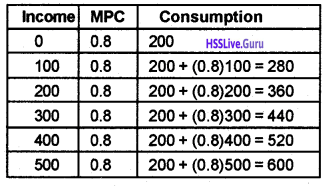Question 4.
“Increase in saving leads to decrease in saving”- comment.
It is usually accepted that an increase in saving leads to a decrease in saving. This is because, if all the people of the economy increase the proportion of income they save (i.e. if the mps of the economy increases) the total value of savings in the economy will not increase – it will either decline or remain unchanged. This result is known as the ‘Paradox of Thrift’ – which states that as people become more thrifty they end up saving less or same as before.

Question 5.
What is deflationary gap? State two measures to remove it.
When aggregate demand falls short of aggregate supply at full employment, it gives rise to deflationary gap. Thus,
deficient demand = aggregate supply – aggregate demand. Measures to remove deflationary gap,

1. Reduce the bank rate.
2. Reduce the cash reservice ratio.

Question 6.
Depict ‘inflationary gap’ in a diagram.
The excess of aggregate demand over aggregate supply at full employment level is known as inflationary gap. The following diagram depicts inflationary gap.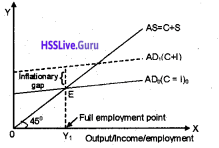Question 7.
What are the components of aggregate demand?
The components of aggregate demand are:

1. Household consumption expenditure
2. Government consumption expenditure
3. Private Investment expenditure

Question 8.
Using data given below construct and aggregate demand schedule for the level of income 500,1000, 1500 and 2000.
$$\overline{\mathrm{C}}=50, \mathrm{c}=0.8, \overline{\mathrm{I}}=60$$
$$A D=\bar{C}+\overline{1}+c \cdot y$$Question 9.
The diagram below shows parametric shifts. Explain parametric shift and identify the reason for shifts in the following diagram.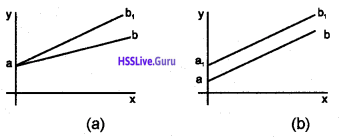parametric shifts are those shifts in the lines of a diagram, due to changes in slope or intercept. In diagram (a) the line shifts upward due to increase in the slope. In diagram (b) the shift in the line is due to the change in the intercept. The intercept increases here.

Question 10.
According to Keynesian model, as saving propensity increases, the equilibrium income decreases. Since the level of income is reduced, the volume of savings also comes down automatically.

1. Can you associate the above statement with any Keynesian proposition? Name it.
2. Substantiate with an example.

2. example.Question 11.
The following diagram shows the equilibrium income situation of a hypothetical economy with mpc 0.6 and a =70Analyse the following situations and represent the resulting change in the diagram.

1. Income of the economy increases due to a good harvest. Consequently, the mpc increased to 0.7
2. Income of the economy increases due to a good harvest. Consequently, the mpc increased to 0.7
3. An amount of 50 million is planned to invest for the construction of a new multipurpose dam in the economy.
4. Calculate the new equilibrium income of the economy.

1. Change in the slope parameter. AD curve swings upward

2. Change in the intercept parameter. AD curve shift upward

3.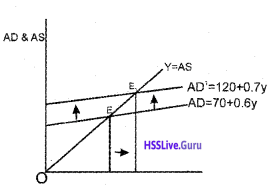### Plus Two Economics Income Determination Five Mark Questions and Answers

Question 1.
Match the following.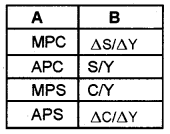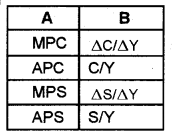Question 2.
From the following data, calculate

1. MPC
2. APC
3. MPS
4. APS

Consumption = 400, Income = 500, Change in income 600, change in consumption = 450
From the data, we get, C = 400,Y = 500, ΔC = 50, ΔY = 100

1. MPC = ΔC/ΔY = 50/100 = 0.5
2. APC = C/Y = 400/500 = 0.8
3. MPS = ΔS/ΔY = 50/100 = 0.5
4. APS = S/Y = 100/500 = 0.2Question 3.
Suppose income increases by 10 and the MPC is 0.8. Explain the multiplier mechanism with the help of a table.
When income increases by 10, consumption expenditure goes up by (0.8)10 since people spend 0.8 (= MPC) fraction of their additional income on consumption. Hence, in the next round, aggregate demand in the economy goes up by (0.8)10 and there again emerges an excess demand equal to (0.8)10.

Therefore, in the next production cycle, producers increase their planned output further by (0.8)10 to restore equilibrium. When this extra output is distributed among factors, the income of the economy goes up by (0.8)10 and consumption demand increases further by (0.8)210, once again creating excess demand of the same amount.

This process goes on, round after round, with producers increasing their output to clear the excess demand in each round and consumers spending a part of their additional income from this extra production on consumption items-thereby creating further excess demand in the next round.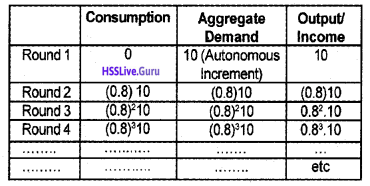In order to find out the total increase in output of the final goods, we must add up the infinite geometric series in the last column, i.e.
10 + (0.8)10 + (0.8)210 + ……… ∝
= 10(1 + (0.8) + (0.8)2 + ……….. ∝}
= 10/1 – 0.8 = 50
The increment in equilibrium value of total output thus exceeds the initial increment in autonomous expenditure. The ratio of the total increment in equilibrium value of final goods output to the initial increment in autonomous expenditure is called the output multiplier of the economy.

Question 4.
Explain the meaning of the break-even point. Illustrate the concept.
Break-even point refers to that point in the level of income at which consumption expenditure becomes exactly equal to income and there is no saving. In other words, whole of income is spent on consumption and as a result, there is no saving.

It happens when income increases from low level to high level, it becomes equal to consumption expenditure at some level and that level is called break even point. Below this level of income, consumption is greater than income but above this level, income is greater than consumption.

This concept can be illustrated as follows. A hypothetical consumption and saving schedule is given.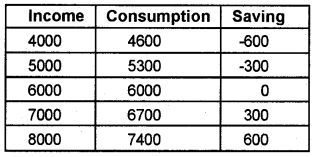Question 5.
State the relationship between MPC and MPS.
1. Marginal Propensity to Consume refers to the ratio of change in consumption to change in income. MPC = AC / AY.

2. Marginal Propensity to Save refers to the ratio of change in saving to change in income. MPS = ΔS / ΔY.

3. The sum of MPC and MPS is always one and equal to unity. That is MPC + MPS = 1 MPS = ΔS / ΔY.

Question 6.
MPC of Indian economy is 0.5 and if the level of investment in the economy is increased by 100.

1. Give the equation for multiplier.
2. Calculate the value of multiplier.
3. If the level of investment declines what will be the effects on total income.

1. The equation for multiplier is, $$k = \frac{1}{1-M P S}$$
2. Since MPC = 0.5, we get the value of multiplier as,
$$K = \frac{1}{1-0.5} = \frac{1}{0.5} = 2$$
3. Since investment is increased by ₹100,
AY = K. Δl ΔY =2 x 100 = 200

When the level of investment declines, the total in-come in the economy will decline.

Question 7.
From the following data, calculate
a) APC
b) APS
c) MPC
d) MPS

• Income = ₹2500
• Consumption = ₹1000
• Change in income = ₹750
• Change in consumption =₹250

In the given example,

• Income = 2500 (Y)
• Consumption = 1000 (C)
• Change in income = 750 (ΔY)
• Change in consumption =750 (ΔC)Question 8.
In an economy investment increases by ₹120 crores. The value of multiplier is 4. Calculate the MPC.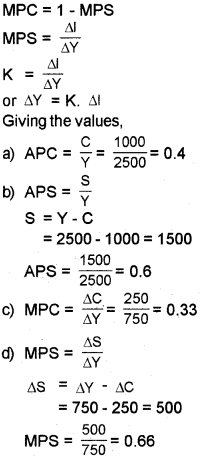Question 9.
In an economy investment increases by ₹120 crore. The value of multiplier is 4. Calculate the MPC.
What do you understand by “parameters of line”? How does a line shifts when its

1. slope increases and
2. its intercept increase?

Consider the equation of a straight line of form b = ma + e where, m 0 is called the slope of the straight line, e is called the intercept on the vertical axis. When a increased by 1 unit the value b increases by m units. These are called movements of variables along the line. The entities e and m are called the parameters of the line. As the value of m increases the straight line swings upwards. This is called a parametric shift of line.

1. A positively sloping straight line swings downward as its slope decreases.
2. A positively sloping straight line shifts upwards parallel when its intercept increases.

Question 10.
What is effective demand? How will you derive the autonomous expenditure multiplier when price of goods and the rate of interest are given?
If the elasticity of supply is infinite, then the output will be solely determined by the aggregate demand at this price in the economy. This called effective demand. The equilibrium output and aggregate demand at the given price of goods and rate of interest is derived by solving the equation.
Y = A + CY
or Y – CY = A
or Y(1 – C) = A
A is the total value of autonomous expenditure. The value of Y is dependent on the value of parameters A and C.Question 11.
State true or falls. Correct if they are wrong.
(a) v = c + s
(b) MPC + MPS ≥ 1
(c) $$K = \frac{1}{1-M P S}$$
(d) ΔY = KΔl
(c) $$K = \frac{1}{1-M P S}$$

Question 12.
Graphically determine the following situations.

1. Full employment situation or equilibrium
2. Deficient demand
3. Excess demand

1. Full employment situation or equilibrium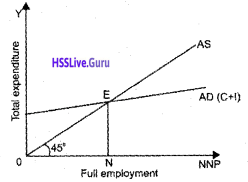2. Deficient demand3. Excess demand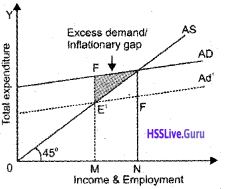Question 13.
Suggest the fiscal policy measures to correct excess demand and deficient demand
a. Excess demand

• Increase taxes
• Decrease Government expenditure
• Reduce deficit financing
• Increase public borrowing

b. Deficient demand

• Decrease taxes
• Increase government expenditure
• Increase deficit financing
• Reduce public borrowing

Question 14.
identify the relation between multiplier and MPC.
The value of the multiplier is determined by marginal propensity to consume. Higher the MPC, greater the size of multiplier lower the MPC, smaller the size of multiplier.

When income of consumers rises they spend more the value of increase in income, i.e., multiplier depends on MPC, greater the value of multiplier depends on greater size of MPC. Thus there is direct relation between multiplier and MPC. The relation can be expressed in terms of an equation as under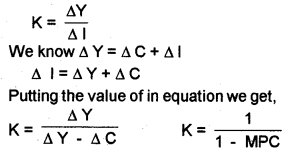Thus it is clear from the above equation that the value of MPC and multiplier are positively related.Question 15.
In the function AD = $$\overline{\mathrm{A}}$$+ c. y what happens to the aggregate demand curves when,

1. $$\overline{\mathrm{A}}$$
2. C (mpc) increases.

1. $$\overline{\mathrm{A}}$$When $$\overline{\mathrm{A}}$$ increases, the AD curve shifts upwards parallel to the original AD curve. As a result, income increases from Y, to Y2 and economy reaches to new equilibrium position E2.
2. C (mpc) increases.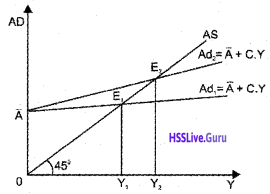When c increases, the AD curve shifts upwards. But it is not a parallel shift, it only swings upward.

Question 16.
Nowadays, all governments of third world economies try to attract domestic and foreign investors by providing financial concessions and establishing Special Economic Zones.

1. Do you think that investment is an essential component of economic growth? Why?
2. Suppose an investor proposed to invest Rs.300crore in an economy with the mpc value of 0.7. Calculate the impact of this investment on the equilibrium income (Multiplier Effect) of the economy?

1. Yes. Investment has a multiplier effect on income. Hence investment is necessary for economic growth.
2. impact of this investment on the equilibrium income### Plus Two Economics Income Determination Eight Mark Questions and Answers

Question 1.
Diagram shows the equilibrium of an economy.

1. Identify the equilibrium level of output and explain it.
2. The diagram show what happens to the equilibrium level of output when investment increases.
3. Analyze the change in investment and the change in output.1. The economy will be in equilibrium when AD = Y1 $$A D=\bar{C} + \bar{I} + c, y$$ The two components of aggregate demand in a two-sector model is consumption and investment. In the diagram, OY is the equilibrium level of output.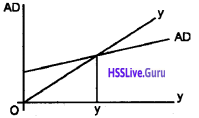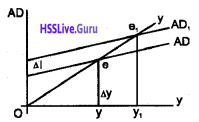$$\text { Multiplier } K = \frac{1}{1-M P C}$$